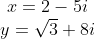## Binomial Product

In polar form, what is the product of the following two binomials?Hint
Use the FOIL ( F irst, O uter, I nner, L ast) method to multiply the two binomials (an expression with two terms). Remember to track +/- signs.
$$(a+b)(c+d)=ac+ad+bc+bd$$$Hint 2 Polar form is: $$z=c\: \angle \: \theta$$$
where $$c=\sqrt{a^2+b^2}$$ (the vector’s length) and $$\theta=tan^{-1}(b/a)$$ . Note $$a$$ is the real component and $$b$$ is the imaginary component of the rectangular form.
Use the FOIL ( F irst, O uter, I nner, L ast) method to multiply the two binomials (an expression with two terms). Remember to track +/- signs.
$$(a+b)(c+d)=ac+ad+bc+bd$$$Thus, $$(2-5i)(\sqrt{3}+8i)=2\sqrt{3}+16i-5\sqrt{3}i-40i^2$$$
Remember $$i=\sqrt{-1}$$ , so we can simplify:
$$=3.46+16i-8.66i-40(-1)$$$$$=3.46+40+7.34i=43.46+7.34i$$$
Complex numbers can be written in both rectangular and polar form. For rectangular form, a complex number is represented by both its real and imaginary components:
$$z=a+jb$$$where $$a$$ is the real component, $$b$$ is the imaginary component, and $$j=\sqrt{-1}$$ which is often expressed as $$i=\sqrt{-1}$$ . Analyzing our rectangular coordinate form $$43.46+7.34i$$ , we can say $$a=43.46$$ and $$b=7.34$$ . Polar form is: $$z=c\: \angle \: \theta$$$
where $$c=\sqrt{a^2+b^2}$$ (the vector’s length) and $$\theta=tan^{-1}(b/a)$$ . Note: $$a=c\:cos(\theta)$$ and $$b=c\:sin(\theta)$$ .
Therefore,
$$c=\sqrt{43.46^2+7.34^2}=\sqrt{1,888.77+53.876}=\sqrt{1,942.65}=44.08$$$$$\theta=tan^{-1}(\frac{7.34}{43.46})=9.6^{\circ}$$$
Finally, the polar form is:
$$44.08 \: \angle \: 9.6^{\circ}$$$$$44.08 \: \angle \: 9.6^{\circ}$$$